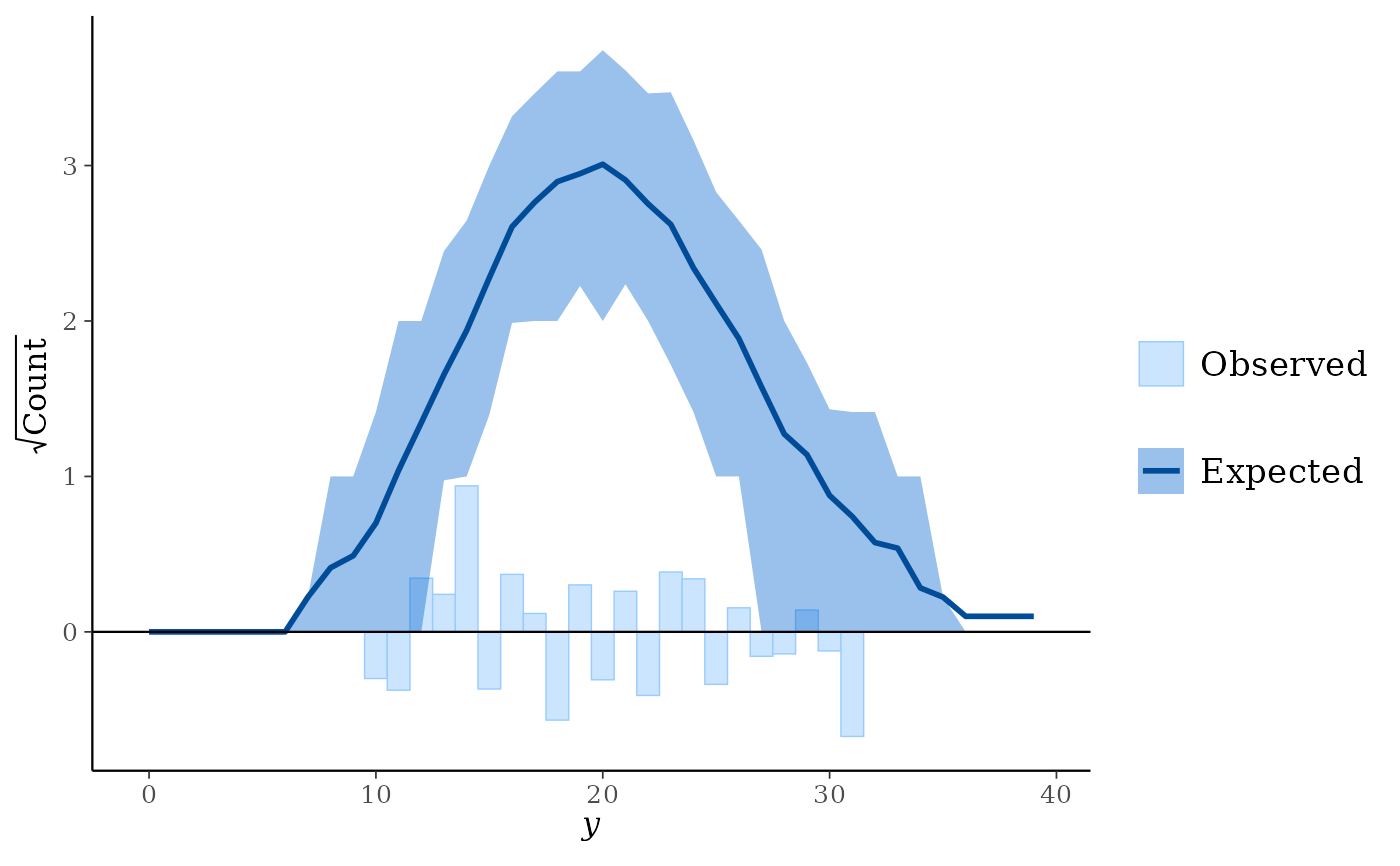Many of the PPC functions in bayesplot can be used with discrete data. The small subset of these functions that can only be used if y and yrep are discrete are documented on this page. Currently these include rootograms for count outcomes and bar plots for ordinal, categorical, and multinomial outcomes. See the Plot Descriptions section below.

ppc_bars(
y,
yrep,
...,
prob = 0.9,
width = 0.9,
size = 1,
fatten = 2.5,
linewidth = 1,
freq = TRUE
)

ppc_bars_grouped(
y,
yrep,
group,
...,
facet_args = list(),
prob = 0.9,
width = 0.9,
size = 1,
fatten = 2.5,
linewidth = 1,
freq = TRUE
)

ppc_rootogram(
y,
yrep,
style = c("standing", "hanging", "suspended"),
...,
prob = 0.9,
size = 1
)

ppc_bars_data(y, yrep, group = NULL, prob = 0.9, freq = TRUE)

## Arguments

y

A vector of observations. See Details.

yrep

An S by N matrix of draws from the posterior (or prior) predictive distribution. The number of rows, S, is the size of the posterior (or prior) sample used to generate yrep. The number of columns, N is the number of predicted observations (length(y)). The columns of yrep should be in the same order as the data points in y for the plots to make sense. See the Details and Plot Descriptions sections for additional advice specific to particular plots.

...

Currently unused.

prob

A value between 0 and 1 indicating the desired probability mass to include in the yrep intervals. Set prob=0 to remove the intervals. (Note: for rootograms these are intervals of the square roots of the expected counts.)

width

For bar plots only, passed to ggplot2::geom_bar() to control the bar width.

size, fatten, linewidth

For bar plots, size, fatten, and linewidth are passed to ggplot2::geom_pointrange() to control the appearance of the yrep points and intervals. For rootograms size is passed to ggplot2::geom_line().

freq

For bar plots only, if TRUE (the default) the y-axis will display counts. Setting freq=FALSE will put proportions on the y-axis.

group

A grouping variable of the same length as y. Will be coerced to factor if not already a factor. Each value in group is interpreted as the group level pertaining to the corresponding observation.

facet_args

An optional list of arguments (other than facets) passed to ggplot2::facet_wrap() to control faceting.

style

For ppc_rootogram, a string specifying the rootogram style. The options are "standing", "hanging", and "suspended". See the Plot Descriptions section, below, for details on the different styles.

## Value

The plotting functions return a ggplot object that can be further customized using the ggplot2 package. The functions with suffix _data() return the data that would have been drawn by the plotting function.

## Details

For all of these plots y and yrep must be integers, although they need not be integers in the strict sense of R's integer type. For rootogram plots y and yrep must also be non-negative.

## Plot Descriptions

ppc_bars()

Bar plot of y with yrep medians and uncertainty intervals superimposed on the bars.

ppc_bars_grouped()

Same as ppc_bars() but a separate plot (facet) is generated for each level of a grouping variable.

ppc_rootogram()

Rootograms allow for diagnosing problems in count data models such as overdispersion or excess zeros. They consist of a histogram of y with the expected counts based on yrep overlaid as a line along with uncertainty intervals. The y-axis represents the square roots of the counts to approximately adjust for scale differences and thus ease comparison between observed and expected counts. Using the style argument, the histogram style can be adjusted to focus on different aspects of the data:

• Standing: basic histogram of observed counts with curve showing expected counts.

• Hanging: observed counts counts hanging from the curve representing expected counts.

• Suspended: histogram of the differences between expected and observed counts.

All of the rootograms are plotted on the square root scale. See Kleiber and Zeileis (2016) for advice on interpreting rootograms and selecting among the different styles.

Other PPCs: PPC-censoring, PPC-distributions, PPC-errors, PPC-intervals, PPC-loo, PPC-overview, PPC-scatterplots, PPC-test-statistics

## Examples

set.seed(9222017)

# bar plots
f <- function(N) {
sample(1:4, size = N, replace = TRUE, prob = c(0.25, 0.4, 0.1, 0.25))
}
y <- f(100)
yrep <- t(replicate(500, f(100)))
dim(yrep)
#>  500 100
group <- gl(2, 50, length = 100, labels = c("GroupA", "GroupB"))

color_scheme_set("mix-pink-blue")
ppc_bars(y, yrep)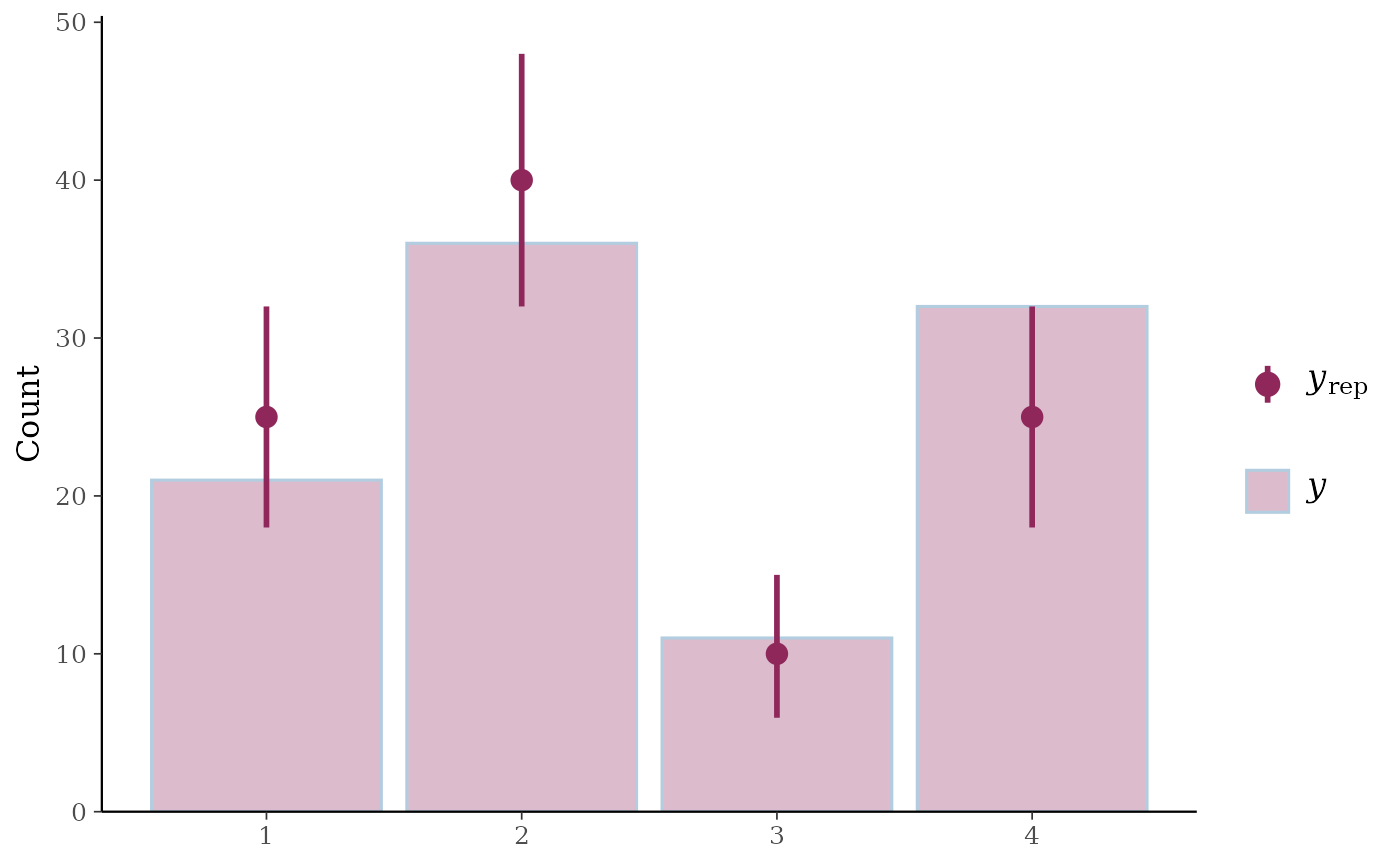# split by group, change interval width, and display proportion
# instead of count on y-axis
color_scheme_set("mix-blue-pink")
ppc_bars_grouped(y, yrep, group, prob = 0.5, freq = FALSE)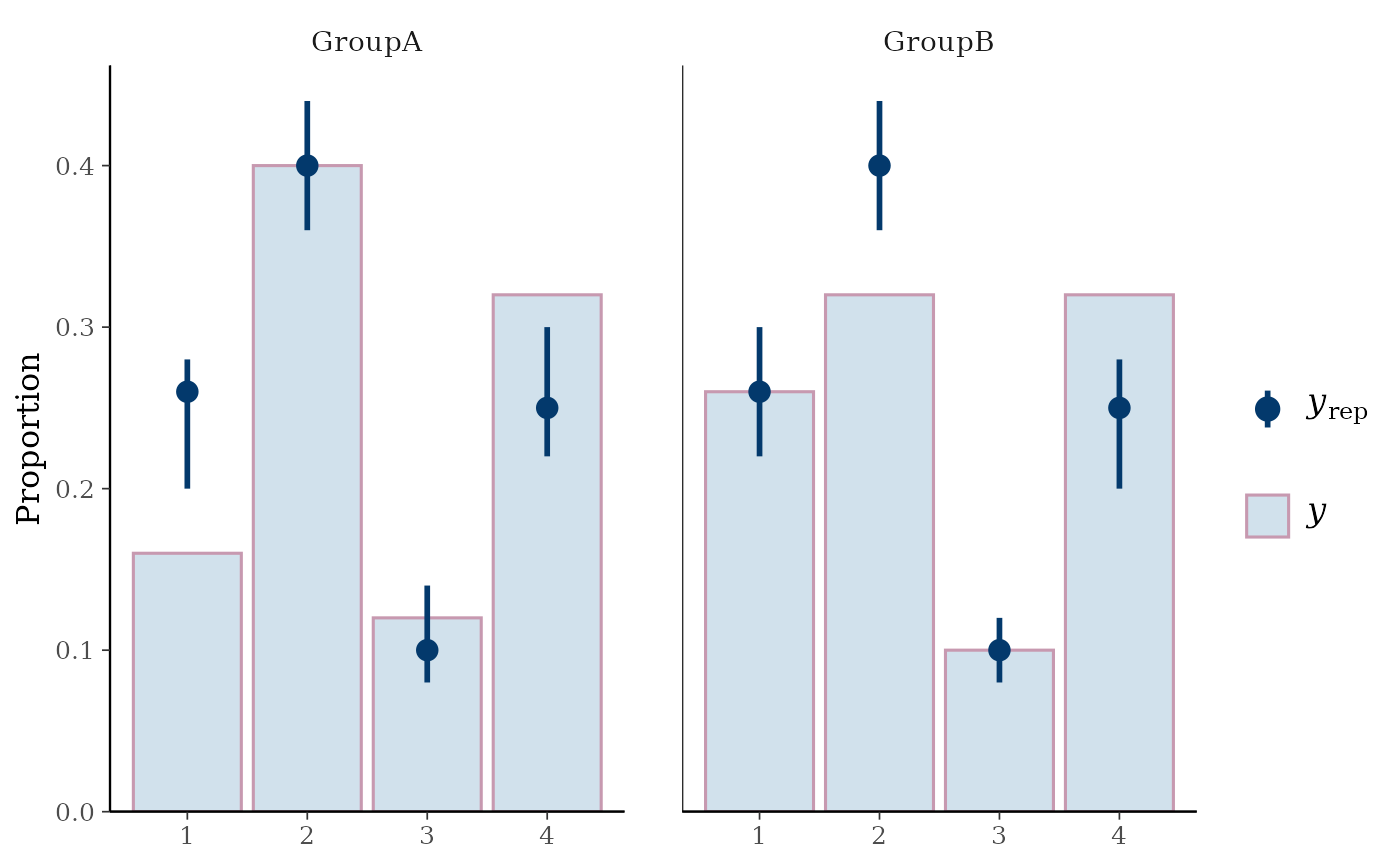# \dontrun{
# example for ordinal regression using rstanarm
library(rstanarm)
fit <- stan_polr(
tobgp ~ agegp,
data = esoph,
method = "probit",
prior = R2(0.2, "mean"),
init_r = 0.1,
seed = 12345,
# cores = 4,
refresh = 0
)

# coded as character, so convert to integer
yrep_char <- posterior_predict(fit)
print(yrep_char[1, 1:4])
#>          1          2          3          4
#> "0-9g/day" "0-9g/day"    "10-19"    "10-19"

yrep_int <- sapply(data.frame(yrep_char, stringsAsFactors = TRUE), as.integer)
y_int <- as.integer(esoph$tobgp) ppc_bars(y_int, yrep_int)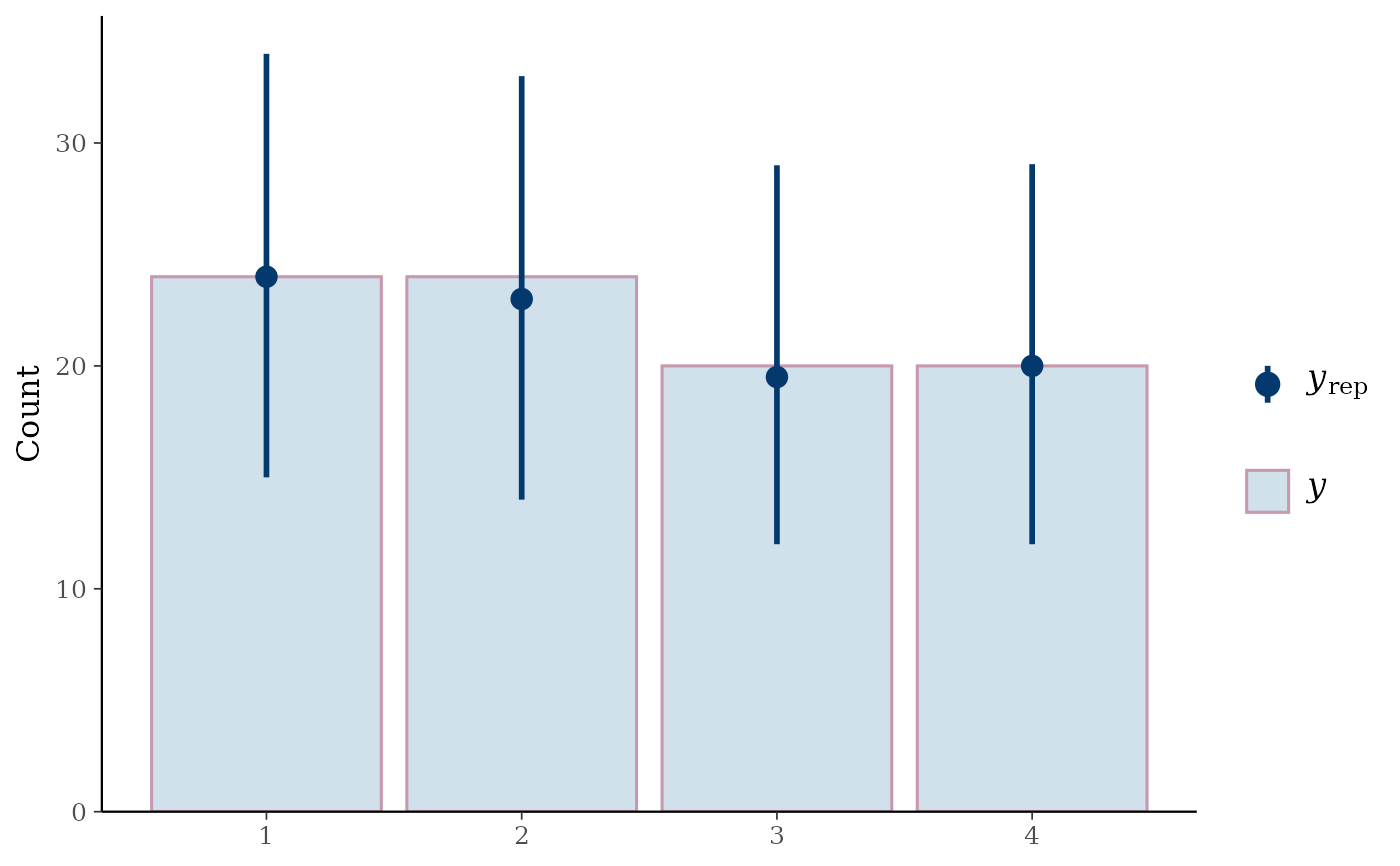ppc_bars_grouped( y = y_int, yrep = yrep_int, group = esoph$agegp,
freq=FALSE,
prob = 0.5,
fatten = 1,
size = 1.5
)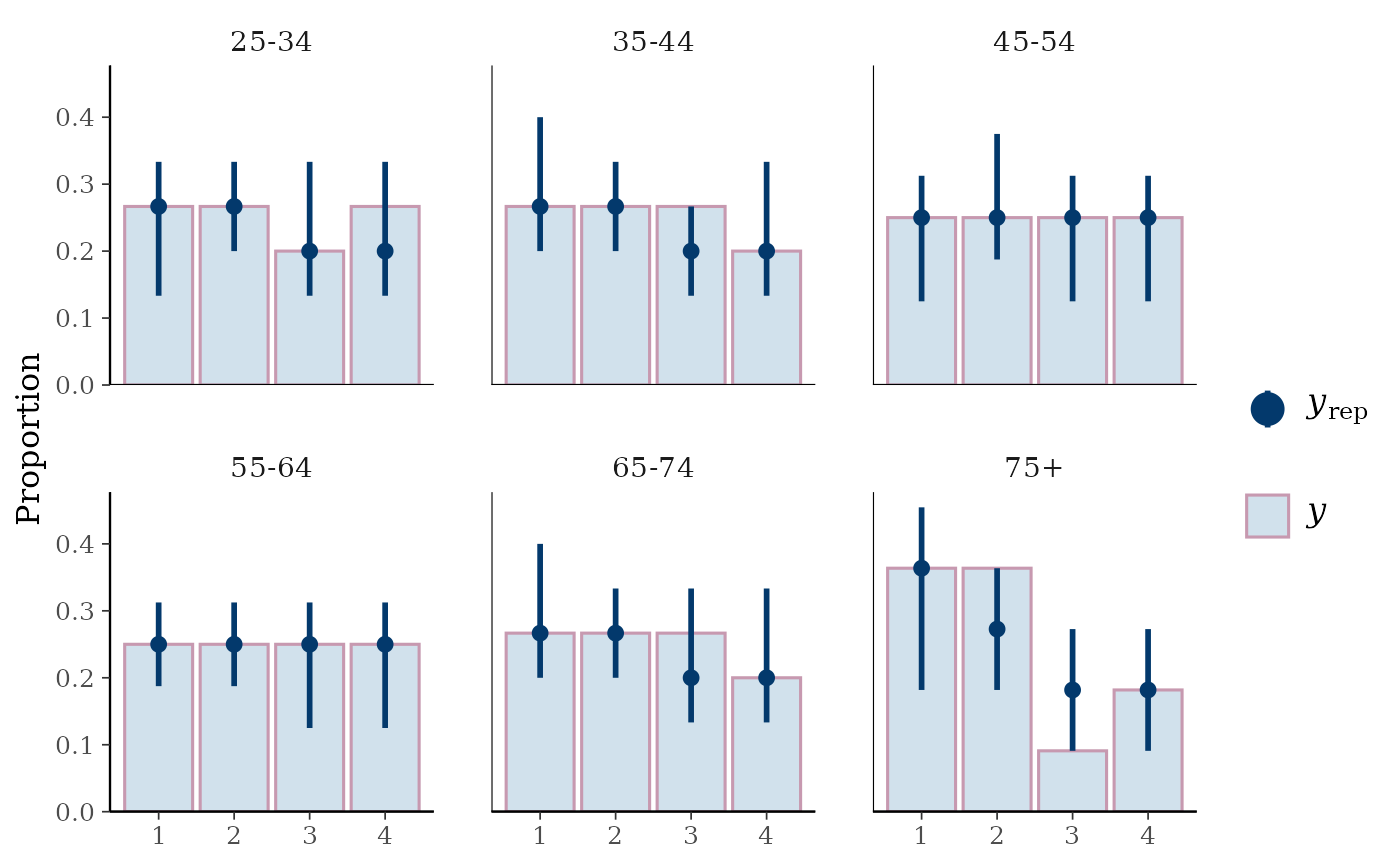# }

# rootograms for counts
y <- rpois(100, 20)
yrep <- matrix(rpois(10000, 20), ncol = 100)

color_scheme_set("brightblue")
ppc_rootogram(y, yrep)ppc_rootogram(y, yrep, prob = 0)ppc_rootogram(y, yrep, style = "hanging", prob = 0.8)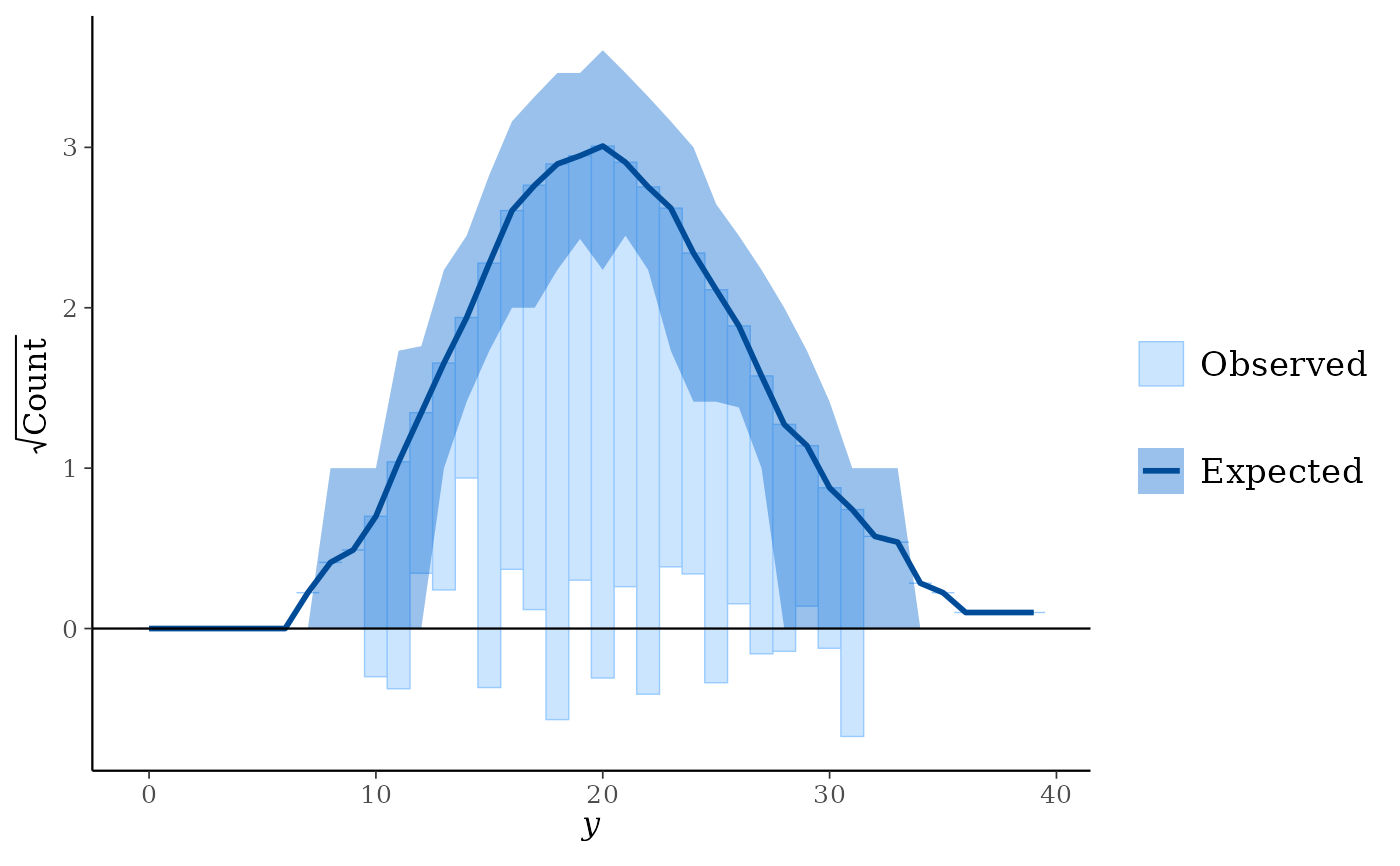ppc_rootogram(y, yrep, style = "suspended")